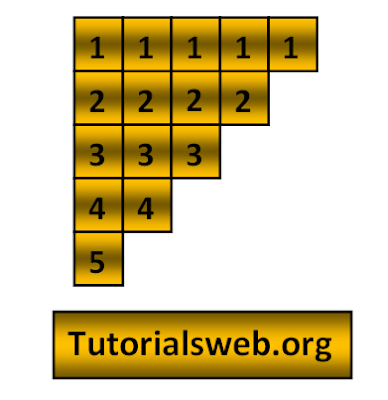## Write "C"  program to print below Number pattern 12:To understand this example, you should have knowledge of the following topics:
·      C programming operators
C Number pattern 12 program using For loop:
`#include <stdio.h>`
`int main()`
`{`
`    int i, j;`
`    for(i=1;i<=5;i++)`
`    {`
`        for(j=5;j>=i;j--)`
`        {`
`            printf("%d",i);`
`        }`
`        printf("\n");`
`    }`
`    return 0;`
`}`
Output:
`11111`
`2222`
`333`
`44`
`5`
Explanation:
• First two integers "i" and "j" are declared of type int.
• Then in the First for loop “i” value is initialized with a value 1.
• Now “i” value is checked with the condition i <= 5 that is (1<= 5). So the condition is True.
• Now the loop enters into second for loop and checks the condition j >= i. Where "j = 5".
• So the value of j=5 is greater than the value of "i" it prints the value if "i". Now the value of "j" is decremented to "4" the second for loop condition becomes True and continues until the condition become False.
• Then the value of "i" is incremented to "2" and repeat the same process.
• Last where “i” value becomes “6” the first for loop condition becomes false and the program terminates.